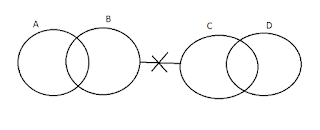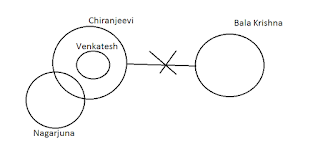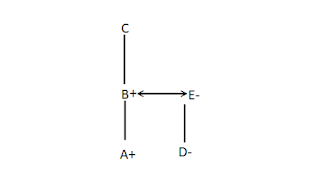# Reasoning Mock Test for RBI Grade B - IAlphanumeric Series is a series of characters which contains Alphabets, Numbers and Symbols. Questions are asked based on given series. In bank exams this type of series can ask with weightage of 5 marks which can be easily doable.

## Alphanumeric series

### Directions (1-10): Study the following arrangement carefully and answer the questions given below:

F A B % 6 K I @ 2 O G E & 9 C V T 7 \$ 3 # 7 R L * 4

### Question no. 1

Which of the following is sixth to the left of 13th from right end?
A) 2
B) O
C) @
D) %
E) I

### Question no. 2

How many symbols are there which are followed by a number and preceded by a consonant?
A) None
B) One
C) Two
D) Three
E) More than three

### Question no. 3

What is the word formed by 3rd, 12th alphabets from left end and 10th alphabet from right end in respective order?
A) BEK
B) BEL
C) BER
D) BET
E) None

### Question no. 4

How many consonants are there which are followed and preceded by vowels?
A) None
B) One
C) Two
D) Three
E) More than three

### Question no. 5

How many vowels are there which are followed by symbol and preceded by a consonant?
A) None
B) One
C) Three
D) More than three
E) Two

### Question no. 6

How many symbols are there which are in between two numbers in which the difference between the numbers is four?
A) None
B) Two
C) More than three
D) One
E) Three

### Question no. 7

Which of the following is exactly between the characters which are 21st and 20th from left end and right end respectively?
A) 9
B) &
C) E
D) V
E) C

### Question no. 8

If all the numbers are removed which of the following is 8th from right end?
A)
O
B) G
C) @
D) \$
E) C

### Question no. 9

If first half and 2nd half of the sequence are interchanged, then which of the following is 13th to the left of O?
A) \$
B) R
C) None of these
D) 9
E) C

### Question no. 10

What is the sum of the numbers which are followed by a consonant and preceded by symbol?
A) 20
B) 21
C) 22
D) 23
E) 24

## SYLLOGISMS

Directions (11-12): In each question below are given some statements followed by some conclusions. You have to take the given statements to be true even if they seem to be at variance with commonly known facts. Read all the conclusions and then decide which of the given conclusions logically follows the given statements disregarding commonly known facts. Give answer-
(a) If only conclusion I follows.
(b) If only conclusion II follows.
(c) If either conclusion I or II follows.
(d) If neither conclusion I nor II follows.
(e) If both conclusions I and II follow.

### Question no. 11

Statements:
1. All tigers are cats.
2. Some tigers are lions.
3. All cats are rats.
Conclusions:
I) Some cats are not tigers.
II) All lions are rats is a possibility.

### Question no. 12

Statements:
1. Some heroes are heroines.
2. No heroine is director.
3. All heroes are producers.
Conclusions:
I) Some directors are producers.
II) No director is a producers.

Directions (13-15): Some statements are given followed by some conclusions. You have to consider the statements to be true even if they seem to be at variance from commonly known facts. You have to decide which of the following conclusions if any, follow from the given statements:

### Question no. 13

Statements:
1. Some A are B.
2. No B is C.
3. Some C are D
Conclusions:
i) Some D are B
ii) All D being B is Possible.
iii) All D is C

A) Only (i) and (iii) follow
B) Only (iii) follow
C) Either (i) or (ii) follow
D) All follow
E) None of the above

### Question no. 14

Statements:
1. No Chiranjeevi is Bala Krishna.
2. All Vekatesh are Chiranjeevi.
3. Some Chiranjeevi are Nagarjuna.
Conclusions:
i) No Vekatesh is Bala Krishna.
ii) Some Venkatesh being Nagarjuna is possible.
iii) All Nagarjuna is Bala Krishna is a possibility.

A) Only (i) follow
B) Only (iii) follow
C) Only (i) and (iii) follow
D) Only (i) and (ii) follow
E) None of the above

### Question no. 15

Statements:
1. Some laptop are computers.
2. No laptop is mobile phone.
3. Some mobile phones are tablet.
Conclusions:
i) No tablet is laptop.
ii) Some computer are mobile phone.
iii) Some tablet are laptop.

A) None follow.
B) Only (i) follow.
C) Only (ii) follow.
D) Only (iii) follow.
E) All follow.

## DATA SUFFICIENCY

Directions (16-20): Each of the following questions below consists of a question and two statements numbered I and II given below it. You have to decide whether the data provided in the statements are sufficient to answer the question. Read both the statements and give the answer.
(A)
if the data in statement I alone are sufficient to answer the question, while the data in statement II alone are not sufficient in answer the question.
(B) if the data in statement II alone are sufficient to answer the question, while the data in statement I alone are not sufficient to answer the question.
(C) if the data in either in statement I alone or in statement II alone are sufficient to answer the question.
(D) if the data in both the statements I and II together are not sufficient to answer the question.
(E) if the data in both the statements I and II are together necessary to answer the question.

### Question no. 16

How A related to C?
Statement I: A is son of B, who is only child of C.
Statement II: D is daughter of E who is mother of A.

### Question no. 17

In which direction is Arun with respect to Farhan?
Statement I: Arun is west to the Balu, who is north to Chandu.
Statement II: Farhan is west of Divakar, who is south of Chandu.

### Question no. 18

How ‘WE’ is coded in the given statements?
Statement I: ‘I LOVE HYDERABAD’ coded as “na, ka, li” and ‘WE LOVE INDIA’ coded as “li, pa, ma” in particular language.
Statement II: ‘INDIA IS BEST’ is coded as “pa, ni, sa” in same language.

### Question no. 19

Tarun, Manoj, Sam, Bhargav, Vikas are sitting according to their heights in ascending order in a row facing north. Who among them is sitting in centre?
Statement I: Sam is taller than Bhargav and Vikas.
Statement II: The number of persons sitting left to the Sam is same as the persons sitting right to him.

### Question no. 20

Six persons A, B, C, D, E, F sitting in a row facing north. What is the position of C with respect to E?
Statement I: Only two persons are sitting to the left of F, who is immediate right of E.
Statement II: C is sitting at an extreme end and right B.

EXPLANATION:
6th to left of 13th from right means 19th from right end. Therefore answer is “@”. Hence option C is answer.

EXPLANATION:
“B % 6, L*4”. Hence option C is answer.

EXPLANATION:
3rd from left is B, 12th from left end is E, 10th from right end is T. So word formed is BET. Hence option D is answer.

EXPLANATION:
“O G E”. Hence option B is answer.

EXPLANATION:
“K I @, G E &”. Hence option E is answer.

EXPLANATION:
Possible sets are “7 \$ 3” and “4 # 7”. 1st set has difference between numbers is equal to 4. Therefore option D is answer.

EXPLANATION:
21st from left end is “#” and 20th from right end is “I”. The character exactly between these two is “9”. Hence option A is answer

EXPLANATION:
Character “C” is 8th from right after removing numbers. Therefore option E is answer.

EXPLANATION:
When 1st half of sequence and 2nd half of sequence are interchanged, then series formed is
9 C V T 7 \$ 3 # 7 R L * 4 F A B % 6 K I @ 2 O G E &
Then 13th to left of O is “R”. Hence option B is the answer.

EXPLANATION:
The sets are “% 6 K”, “& 9 C”, “# 7R”. So the sum is 6+9+7= 22. Hence option C is answer.

EXPLANATION:EXPLANATION:EXPLANATION:EXPLANATION:EXPLANATION :EXPLANATION :
Both statements are required to answer.EXPLANATION :
Both statements are not sufficient. As distance between them are not known, Arun can be in North or North West or north East w.r.t Farhan.EXPLANATION :
From statement I, LOVE can be coded as “li’. From statement I & II INDIA can be coded as “pa’. From statement I WE is coded as ‘ma’.

EXPLANATION :
From statement I, Sam is sitting in centre as he taller than only Bhargav and Vikas in 5 persons sitting in a row.
From statement II, Sam is sitting in centre.

EXPLANATION :
From Statement I
____ E F ____ ____ ____.
From stamen II
____ ____ ____ ____ ____ C. As C is sitting at extreme end. He may sit at left end or right end. If he sit at left end, then C cant sit to the right of B. So C sit at right end.
From both statements
____ E F ____ ____ C.
Therefore, C is fourth to the right of E.